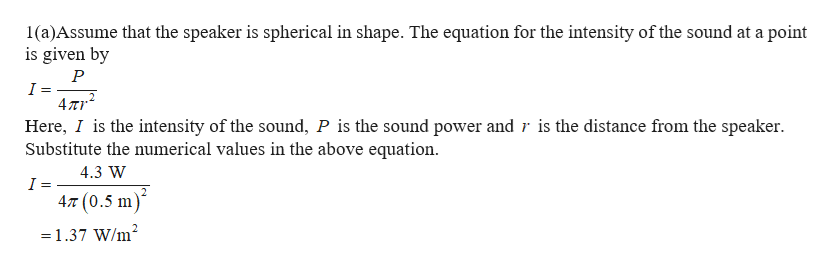# 1a. A speaker is playing music at 4.3 W. What is the intensity of the music if the speaker is 0.5 m away from you?          1b. What is the loudness of this speaker?         Speaker A is playing a sound with double the intensity of Speaker B. How much louder is Speaker A than Speaker B?       Speaker A is playing a sound with a quarter of the amplitude of Speaker B. How much louder is Speaker A than Speaker B?    What is the impedance of a wave with a speed of 243m/s and a density of 3.2 kg/m3.        What is the ratio of impedance when Z1 = 3.2 x 103 and Z2 = 1.45 x 102 ?          7a. The ratio of impedance of two substances is 7. What percentage of intensity is transmitted at the boundary?            7b. What percentage of intensity is reflected?

Question
60 views

1a. A speaker is playing music at 4.3 W. What is the intensity of the music if the speaker is 0.5 m away from you?

1b. What is the loudness of this speaker?

1. Speaker A is playing a sound with double the intensity of Speaker B. How much louder is Speaker A than Speaker B?

1. Speaker A is playing a sound with a quarter of the amplitude of Speaker B. How much louder is Speaker A than Speaker B?

1. What is the impedance of a wave with a speed of 243m/s and a density of 3.2 kg/m3.

1. What is the ratio of impedance when Z1 = 3.2 x 103 and Z2 = 1.45 x 102 ?

7a. The ratio of impedance of two substances is 7. What percentage of intensity is transmitted at the boundary?

7b. What percentage of intensity is reflected?

check_circle

Step 1help_outlineImage Transcriptionclose1(a)Assume that the speaker is spherical in shape. The equation for the intensity of the sound at a point is given by I 47r Here, I is the intensity of the sound, P is the sound power and r is the distance from the speaker Substitute the numerical values in the above equation 4.3 W 4,T (0.5 m) =1.37 W/m2 fullscreen

### Want to see the full answer?

See Solution

#### Want to see this answer and more?

Solutions are written by subject experts who are available 24/7. Questions are typically answered within 1 hour.*

See Solution
*Response times may vary by subject and question.
Tagged in

### Wave Motion